FEATool Multiphysics  v1.16.1 Finite Element Analysis Toolbox
Solvers

This section describes the different physics solvers available with the FEATool Multiphysics toolbox. Physics solvers compute numerical approximations to equations and boundary conditions with discretization schemes such as FDM/FEM/FVM. This results in sparse matrix systems which are subsequently solved (inverted) to yield discrete and approximate numerical solutions to the equations.

FEATool features integrated GUI and CLI interfaces to the linear and non-linear stationary (solvestat), time-dependent (solvetime), and eigenvalue (solveeig) multiphysics solvers. In addition, interfaces to external solvers, such as the Computational Fluid Dynamics (CFD) solvers OpenFOAM and SU2, and the FEA solver FEniCS is also built-in.

The external solver interfaces allow for setting up and defining multiphysics problems directly in MATLAB with the FEATool GUI and CLI interfaces, then export and solve them with the external solvers. Afterwards, the solutions can automatically be imported into the FEATool GUI for further post-processing. Moreover, the multi-simulation solver approach employed by FEATool conveniently allows switching between several solvers for the same problem with one-click, this can be very useful when comparing and validating results and solutions.

# Solve Mode

After geometry, grid, and equation and boundary conditions have been defined one can switch to solve mode by either pressing the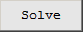mode button, or by selecting Solve Mode in the Solve menu. The solve mode toolbar buttons and menu actions are the following

•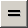Call the default solver as defined by the current solver settings with initial conditions as prescribed by the equation settings. After the problem has been solved FEATool will automatically switch to postprocessing mode and plot the computed solution.
•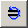Solves the problem using the last computed solution as initial value. Note that for this to work the geometry, grid, physics modes, and finite element shape functions must all remain the same.
•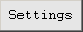Opens the Solver Settings dialog box, alternatively one can also select Solver Settings from the Solve menu.
•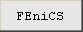Solves multiphysics problems with the external FEA solver FEniCS.
•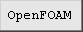Solves incompressible Navier-Stokes, swirl flow, or inviscid compressible Euler fluid flow problems with the external CFD solver OpenFOAM.
•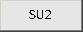Solves incompressible Navier-Stokes or inviscid compressible Euler fluid flow problems with the external CFD solver SU2.
• The Solve Hook... option in the Solve menu opens a dialog box where one can specify solver hook function names.
• Selecting Get Initial Solution from the Solve menu computes the expressions defined in the Initial Condition fields of the equation settings dialog box, and returns this as the solution.

# Multiphysics Solver

The built-in solvers allow for solving coupled stationary, time-dependent, and eigenvalue/frequency multiphysics problems, for which solver settings and parameters and are discussed in the following.

## Solver Settings

The Solver Settings control panel and dialog box shown below can be opened by pressing the correspondingtoolbar button or by selecting Solver Settings... in the Solve menu. The solver settings dialog box allow selecting and configuring the built-in multiphysics solvers.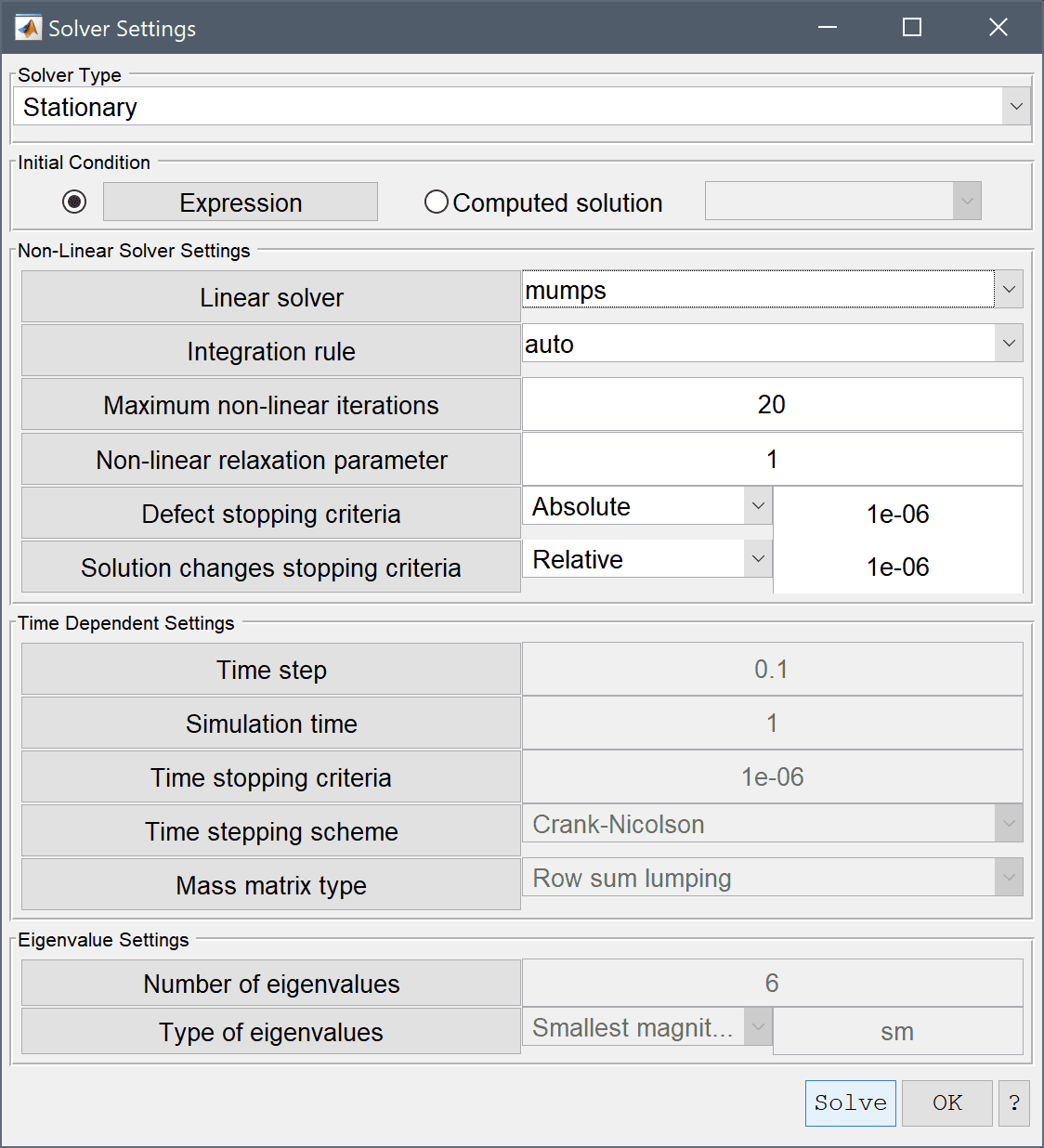### Solver Type

The Solver Type drop-down box allows for either of the

• Stationary - for linear/non-linear static problems (solvestat)
• Time-Dependent - for instationary problems (solvetime)
• Eigenvalue - for eigenvalue/frequency problems (solveeig)

solvers to be selected. The built-in multiphysics solvers are monolithic, meaning that all equations and dependent variables are discretized and solved together in a large coupled system, rather than iteratively solving a segregated set of smaller decoupled systems. This typically allows for faster and more robust convergence behavior at the expense of higher memory usage.

### Initial Condition

The Initial Condition setting controls whether initial values should be computed from Expressions (from the equation settings initial value fields), or use a previously Computed solution. In the latter case the initial solution can be selected from the corresponding list box. Note that for this to work the geometry, grid, physics modes, and finite element shape functions must all remain the same.

### Non-Linear Solver Settings

The Linear solver used to solve sparse matrix systems can be selected from the following options

The default linear solver option is to use either the built-in direct solver (MATLAB's backslash operator) or, alternatively the direct solver mumps which can be noticeably faster and more efficient for many problems. Direct solvers are very robust and fast for a wide range of problems, but are not as memory efficient as iterative solvers such as gmres, bicgstab, or amg. The algebraic multigrid amg solver is experimental, but when it works it potentially can combine the best of solving speed with memory efficiency. Users can also incorporate their own or custom linear solvers by overloading or editing the solvelin function.

The Integration rule or numerical quadrature order used to numerically approximate equation integrals is automatically set to 1 + max(FEM shape function order) by default, but can also be set manually between 1st to 5th order if desired.

A fixed point/Newton non-linear solution process is automatically used when dependent variables are detected in equations and/or boundary coefficients. The Non-Linear Solver Settings allows control over the Maximum number of non-linear iterations, and the Non-linear relaxation parameter which is the fraction of the new to old solution to weigh in to the next non-linear iteration. The non-linear solver will terminate and stop when both the Defect stopping criteria and Solution changes stopping criteria are met which can be set to be measured in either absolute or relative error norms.

### Time Dependent Settings

The Time Dependent Settings control time-stepping parameters when using a time-dependent solver. Time step sets the time step size for the time-stepping schemes. Time dependent simulations will terminate when either the accumulated time has reached the specified Simulation time, or the normed changes in the dependent solution variables are smaller than the Time stopping criteria, which would indicate a stationary state.

The Time stepping scheme setting allows choosing between the following schemes

• Backward Euler - first order implicit
• Crank-Nicolson - second order implicit

The default Crank-Nicolson scheme works well in most situations, for very non-linear or sensitive time-stepping problems one can use the backward Euler method which is more robust but less accurate (to compensate smaller time steps can be used).

Lastly, one can choose between using the Full mass matrix, Row row-sum lumped (default), Diagonal lumped, or consistent HRZ-lumped Mass matrix type representations.

### Eigenvalue Settings

In the Eigenvalue Settings frame one can control the Number of eigenvalues to be computed, as well as the Type of eigenvalues to find (smallest/largest or a search region around a specified eigenvalue/frequency).

## Solver Hooks

Solver hooks allow direct access to, and modification of, the finite element problem struct and matrices before and after linear solver calls in both the stationary (solvestat) and time-dependent (solvetime) solution processes. This enables implementation of non-standard equations and boundary conditions, such as for example periodic and non-axis aligned slip boundary conditions. Two classes of solver hook functions are available, general and boundary hooks.

### General Solver Hooks

To define a general solver hook a function must first be created (here it is for example named fname, and a corresponding m-file function must exist in the MATLAB paths or current working directory). To be activated the function name must be entered in the "Solver Hooks" dialog box (which is opened when selecting Solver Hooks... in the Solve menu), which corresponds to the fea.sol.settings.hook field when modeling using the CLI and scripting interfaces instead of the GUI.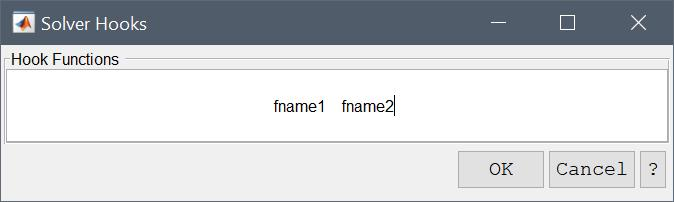The solver hook function must have the following call signature

[ A, f, fea ] = fname( A, f, fea, solve_step )


where the assembled system matrix is A, right hand side/load vector f, and the finite element problem definition struct fea. The solve_step parameter indicates if the function call is directly before the linear solver call (1), or after (2) to manually clean-up any modifications to A, f, or fea if necessary.

### Boundary Solver Hooks

To define a boundary solver hook a Neumann boundary condition must similarly be assigned with the argument solve_hook_fname where fname is the name of the custom function to be called. The boundary hook function must have a function signature of the form

function [ A, f, fea ] = fname( A, f, fea, i_dvar, j_bdr, solve_step )


where again A is the sparse matrix, f the right hand side vector, fea the finite element problem struct, i_dvar dependent variable index, j_bdr boundary number, and solve_step equal to (1) after matrix assembly (but before the linear solver call) and (2) after the linear solver call.

### Solver Hook Example

A simple use of solver hooks can be to monitor the solution process. The example below implements a function to monitor the temperature in a specific point

function [ A, f, fea ] = monitor( A, f, fea, solve_step )
persistent h_fig T
if( isempty(h_fig) )
h_fig = figure( 'Name', 'Custom Solver Monitor' );
end
if( solve_step == 1 )
return
end

T = [ T, evalexpr( 'T', [0.06;0.06], fea ) ];

clf
plot( 1:length(T), T )
grid on
xlabel( 'Iteration' )
ylabel( 'Temperature, T(0.06,0.06)' )
drawnow


To see it in action, save the function in a file named monitor.m, then enter the function name monitor in the Solver Hooks dialog box, and run the Shrink Fitting of an Assembly model example.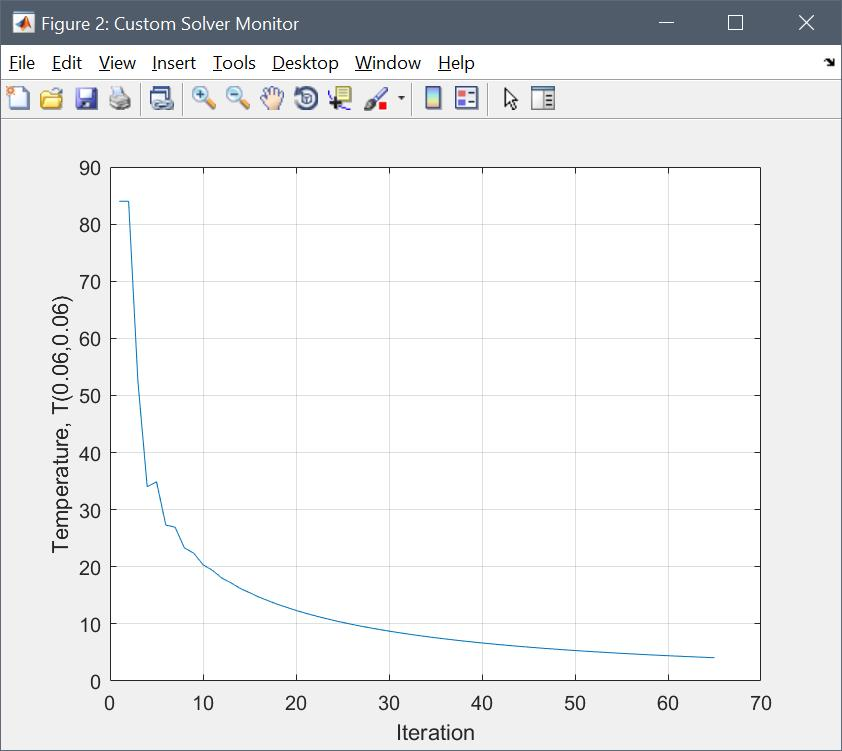For other examples of using solver hooks see the following MATLAB m-file script examples

## Solver Monitors

It is also possible to define custom solver monitoring functions by creating a m-script function with the name fea_solve_monitor.m. If present and detected by MATLAB the function will be called by the built-in solvers after each iteration or time-step with the following function signatures

function [ halt ] = fea_solve_monitor( fea, it )      % for solvestat
function [ halt ] = fea_solve_monitor( fea, tlist )   % for solvetime


where fea is the finite element problem struct, it the non-linear iteration number, and tlist the list of time steps corresponding to the number of columns in the solution vector stored in fea.sol.u. The output argument halt is a logical scalar which indicates if the solution process should be terminated early. For example, the following function for the Shrink Fitting of an Assembly model example will monitor and plot the temperature, T, as a function of time at the point (0.06, 0.06), and stop the solution process when the temperature has reached 15 degrees or lower.

function [ halt ] = fea_solve_monitor( fea, tlist );
persistent h_fig
if( isempty(h_fig) )
h_fig = figure( 'Name', 'Custom Solver Monitor' );
end

for i=1:length(tlist)
T(i) = evalexpr( 'T', [0.06;0.06], fea, i );
end

clf
plot( tlist, T )
grid on
title( ['T@t = ',num2str(tlist(end))])
xlabel( 'Time [s]' )
ylabel( 'Temperature, T(0.06,0.06)' )
drawnow

if( T(end) <= 15 )
halt = true;
else
halt = false;
end


# External Solvers

OpenFOAM CFD Solver

SU2 CFD Solver

FEniCS FEA Solver

# Fluid-Structure Interaction (FSI) Solver

Fluid-structure Interaction (FSI) problems feature a dedicated solver (fsisolve) which handles the physics coupling between the fluid and solid domains, and allows for movement of the grid by using a solid extension mesh motion technique with Jacobian-based stiffening .

The FSI solver supports time-dependent problems with either fully-implicit or semi-implicit discretizations (with explicit geometry treatment). The Initial Condition section allows for either initial conditions from a pre-defined constant or expression, or interpolated from a previously computed solution.

The Time Dependent Settings section allows for prescribing the Initial time, Time step size, and Final time. Specific Output times can also be supplied as a vector of specific times to save and output (default [t0:tstep:tend]). Moreover, the order and accuracy of the employed time discretization schemes can also be selected (1st to 5th for the fluid domain using a Backward Difference Formula (BDF) discretization, and Newmark scheme for the solid domain).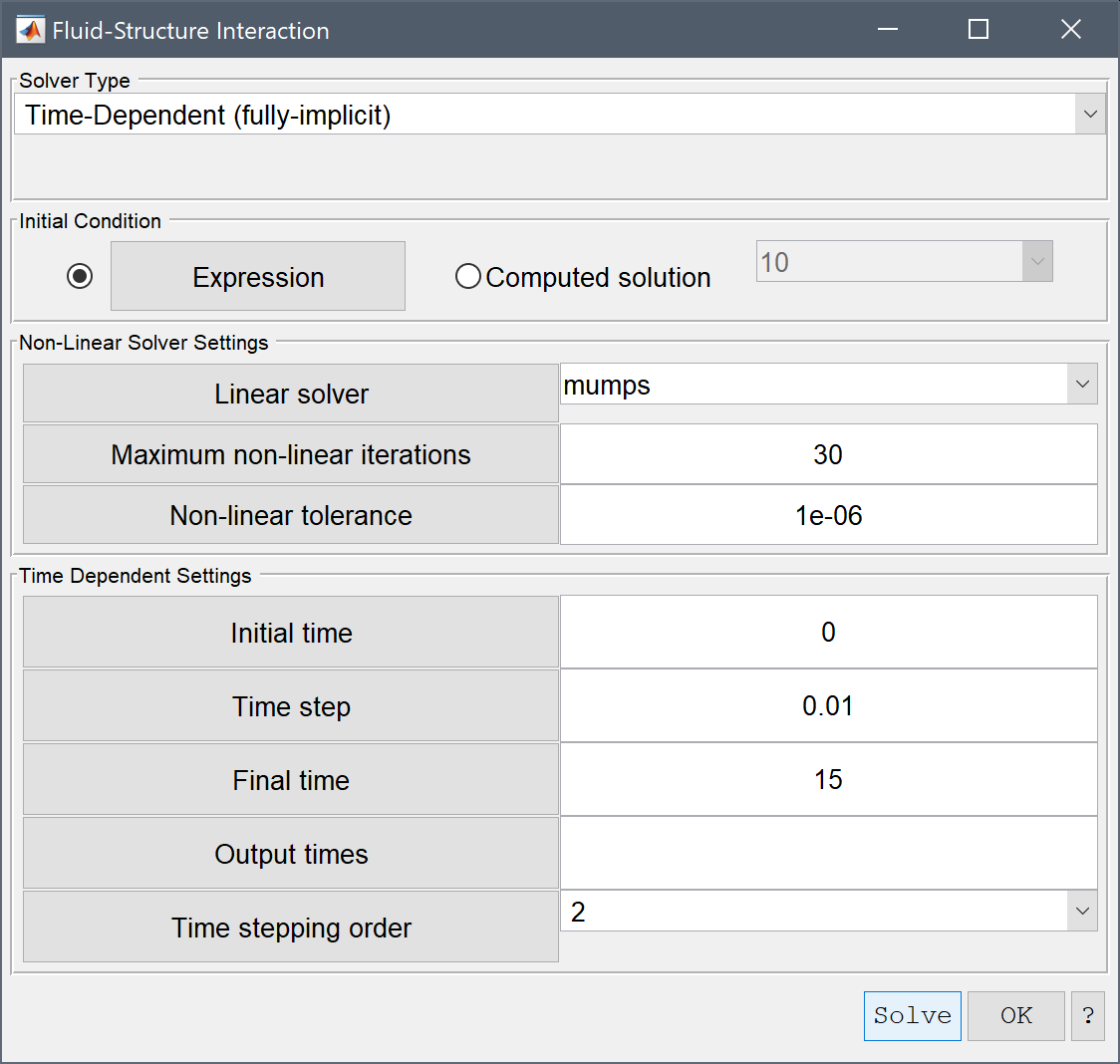A. Quarteroni, A. Manzoni, F. Negri. Reduced Basis Methods for Partial Differential Equations. An Introduction, Springer, 2016.

# Command Reference

The following functions can be used on the MATLAB command line interface for to solve FEATool Multiphysics simulation problems.

Function Description
fenics Solve problem with the external FEniCS solver
fenics_data Convert FEATool problem to FEniCS
openfoam Solve problem with the external OpenFOAM solver
turbulence_inletbccalc Calculate turbulence quantities at inlets
su2 Solve problem with the external SU2 solver
fsisolve Solver for fluid-structure interaction problems
solvelin Wrapper around linear sparse Ax=b system solvers
solveeig Solve eigenvalue/frequency problem
solvestat Solve stationary problem
solvetime Solve time-dependent/instationary problem